Test: Circles- 2

# Test: Circles- 2

Test Description

## 25 Questions MCQ Test Online MCQ Tests for Class 9 | Test: Circles- 2

Test: Circles- 2 for Class 9 2022 is part of Online MCQ Tests for Class 9 preparation. The Test: Circles- 2 questions and answers have been prepared according to the Class 9 exam syllabus.The Test: Circles- 2 MCQs are made for Class 9 2022 Exam. Find important definitions, questions, notes, meanings, examples, exercises, MCQs and online tests for Test: Circles- 2 below.
Solutions of Test: Circles- 2 questions in English are available as part of our Online MCQ Tests for Class 9 for Class 9 & Test: Circles- 2 solutions in Hindi for Online MCQ Tests for Class 9 course. Download more important topics, notes, lectures and mock test series for Class 9 Exam by signing up for free. Attempt Test: Circles- 2 | 25 questions in 25 minutes | Mock test for Class 9 preparation | Free important questions MCQ to study Online MCQ Tests for Class 9 for Class 9 Exam | Download free PDF with solutions
 1 Crore+ students have signed up on EduRev. Have you?
Test: Circles- 2 - Question 1

### AB is a chord of a circle with radius ‘r’. If P is any point on the circle such that ∠APB is a right angle , then AB is equal to

Test: Circles- 2 - Question 2

### In the given figure, AC is a diameter of the given circle and ∠BCD = 75o. Then, ∠EAF−∠ABC is equal to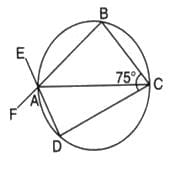Detailed Solution for Test: Circles- 2 - Question 2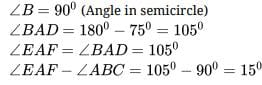Test: Circles- 2 - Question 3

### The region between chord and either of the arc is called

Test: Circles- 2 - Question 4

P is a point on the diameter AB of a circle and CD is a chord perpendicular to AB. If AP = 4 cm and PB = 16 cm, the length of chord CD is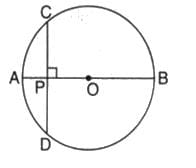Test: Circles- 2 - Question 5

Arc ABC subtends an angle of 130o at the centre O of the circle. AB is extended to P. Then ∠CBP equals :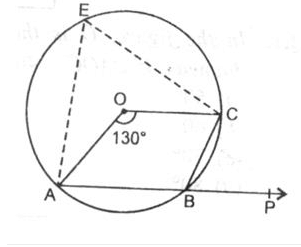Test: Circles- 2 - Question 6

Chords AB and CD intersect at right angles. If ∠BAC = 40o, then ∠ABD is equal to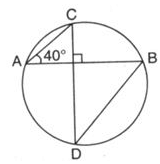Test: Circles- 2 - Question 7

In the given figure, O is the centre of the circle. ∠OAB and ∠OCB are 40o and 30o respectively. Then, the measure of ∠AOC is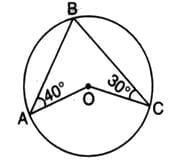Detailed Solution for Test: Circles- 2 - Question 7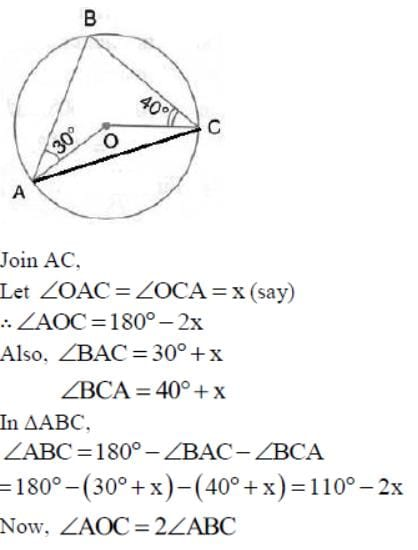(Angle at the centre is double the angle at the circumference subtended by the same chord)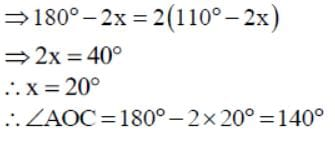Test: Circles- 2 - Question 8

In the given, AB is side of regular five sided polygon and AC is a side of a regular six sided polygon inscribed in the circle with centre O. AO and CB intersect at P, then ∠APB is equal to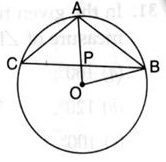Test: Circles- 2 - Question 9

In the given figure, if ∠AOB = 80o and ∠ABC = 30o , then ∠CAO is equal to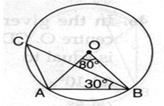Detailed Solution for Test: Circles- 2 - Question 9

2ACB=AOB
ACB=40
CAB+ACB+ABC=180
CAB=180-70
CAB=110
OAB=OBA

AOB+OAB+OBA=180
2OBA=100
OBA=50

CAO=CAB-OBA=110-5O
CAO=60o

Test: Circles- 2 - Question 10

In the figure, O is the centre of eh circle and ∠AOB = 80o. The value of x is :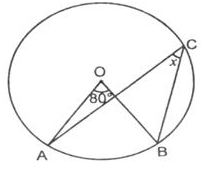Test: Circles- 2 - Question 11

In the given figure if OA = 5 cm, AB = 8 cm and OD is perpendicular to AB, then CD is equal to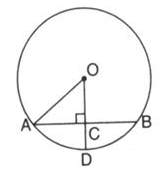Test: Circles- 2 - Question 12

If ∠OAB = 40o, then the measure of ∠ACB is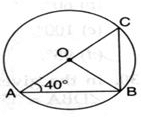Test: Circles- 2 - Question 13

Chords AD and BC intersect each other at right angles at point P. ∠DAB = 35o, then ∠ADC is equal to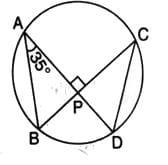Test: Circles- 2 - Question 14

In the given figure, AB is a diameter of the circle APBR. APQ and RBQ are straight lines. If ∠A = 35o and , then the measure of ∠PBR is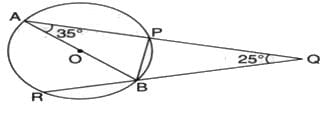Test: Circles- 2 - Question 15

In the figure, O is the center of the circle. If ∠OAB = 40o, then ∠ACB is equal to :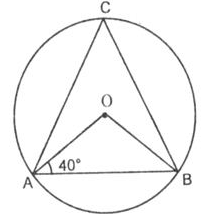Test: Circles- 2 - Question 16

X is a point on a circle with centre O. If X is equidistant from the two radii OP, OQ, then arc PX : arc PQ is equal to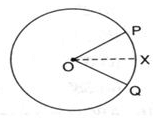Detailed Solution for Test: Circles- 2 - Question 16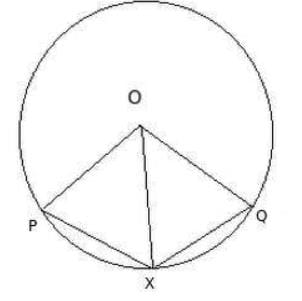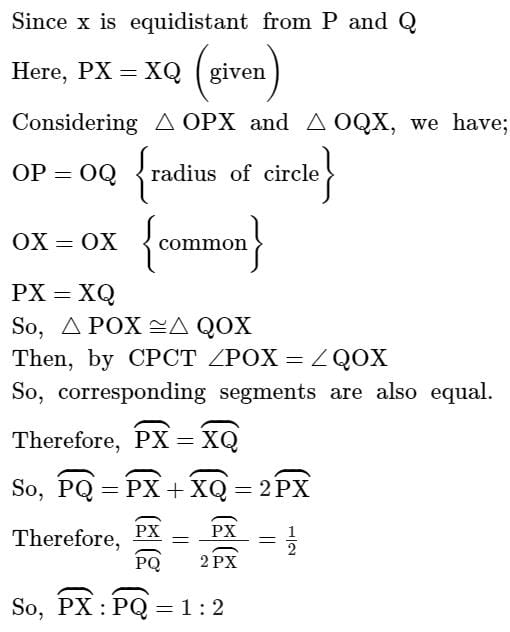Test: Circles- 2 - Question 17

BC is a diameter of the circle and ∠BAO = 60o . Then ∠ADC is equal to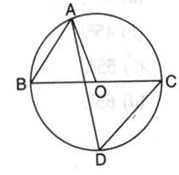Test: Circles- 2 - Question 18

What fraction of the whole circle is minor arc RP in the given figure ?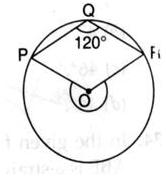Test: Circles- 2 - Question 19

In the given figure, AD is the diameter of the circle and AE = DE. If ∠ABC = 115o, then the measure of ∠CAE is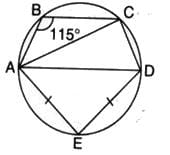Test: Circles- 2 - Question 20

In the figure, if ∠DAB = 60o, ∠ABD = 50o, then ∠ACB is equal to :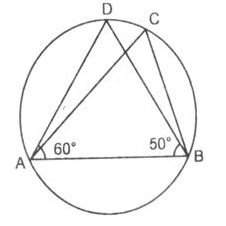Test: Circles- 2 - Question 21

If ABCD is a cyclic trapezium in which AD ║ BC and ∠B = 60o, then ∠BCD is equal to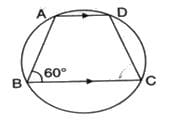Test: Circles- 2 - Question 22

In the given figure, O is the centre of the circle and ∠AOC = 130o. Then ∠ABC is equal to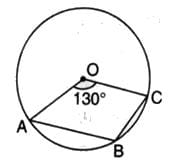Detailed Solution for Test: Circles- 2 - Question 22

360o - 130o =230o

Therefore, angle ABC =1/2reflex angle AOC

Angle ABC =1/2 230o

So, angle ABC =115o

Test: Circles- 2 - Question 23

In the given circle, O is the centre and ∠BDC = 42o. Then, ∠ACB is equal to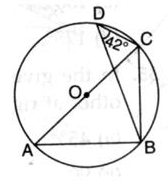Detailed Solution for Test: Circles- 2 - Question 23

In ∆ BDC and ∆ BAC
Angle BAC = BDC
(angle made on same segment BC)
Since ABC is making right angle (90)
So,
In ∆ABC
ABC +BAC+ACB=180
(angle sum property of triangle)
90+42+ACB=180
ACB=180-132
ACB=48o

Test: Circles- 2 - Question 24

AOB is the diameter of the circle. If ∠AOE = 150o, then the measure of ∠CBE is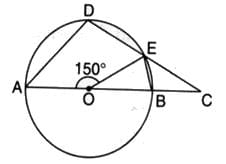Test: Circles- 2 - Question 25

In the given figure, a circle is centred at O. The value of x is :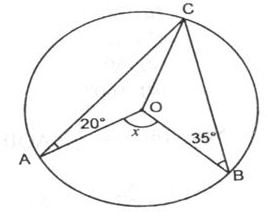## Online MCQ Tests for Class 9

453 tests
 Use Code STAYHOME200 and get INR 200 additional OFF Use Coupon Code
Information about Test: Circles- 2 Page
In this test you can find the Exam questions for Test: Circles- 2 solved & explained in the simplest way possible. Besides giving Questions and answers for Test: Circles- 2, EduRev gives you an ample number of Online tests for practice

453 tests